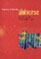© CAMBRIDGE UNIVERSITY PRESS 1999

### 4. THE BARYON FRACTION IN CLUSTERS

Rich clusters of galaxies provide the best laboratory for studying the baryon fraction (i.e., the ratio of the mass in baryons to the total mass of the system) on relatively large scales of ~ Mpc. The mass of baryons in clusters is composed of at least two components: the hot intracluster gas (Section 3) and the luminous parts of the galaxies.

The baryon fraction in clusters is therefore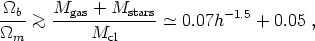(46)

where the first term on the right-hand side represents the gas mass ratio (Section 3.5) and the second term corresponds to the stellar (luminous) contribution.

The baryon density required by big-bang nucleosynthesis is (Walker et al. 1991)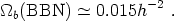(47)

Comparison of the above relations indicates that ifm = 1 then there are many more baryons observed in clusters than allowed by nucleosynthesis. In fact, combining the two relations yields anm value for the mass-density of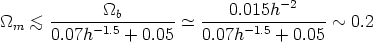(48)

for the observed range of h ~ 0.5-0.8. Therefore, the baryon density given by nucleosynthesis and the high baryon content observed in clusters (mainly in the hot intracluster gas) suggest thatm0.2. This assumes, as expected on this large scale (and as seen in simulations), that the baryons are not segregated relative to the dark matter in clusters (White et al. 1993).

Figure 2 compares the observed gas mass fraction in clusters (baryon fraction) with expectations from cosmological simulations ofm = 1 andm = 0.45 cold-dark-matter (CDM) flat models (Lubin et al. 1996). The results show, as expected, that them = 1 model predicts a much lower gas mass fraction than observed, by a factor of ~ 3. A low-density CDM model withm ~ 0.2-0.3 (in mass) best matches the data, as expected from the general analysis discussed above (see White et al. 1993; White and Fabian 1995; Lubin et al. 1996).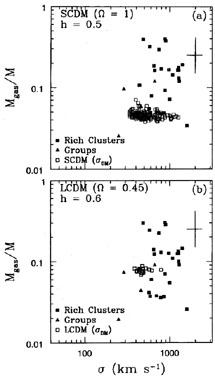Figure 2. Observed and simulated gas mass fraction (Mgas/M) vs. line-of-sight velocity dispersion for rich clusters (Lubin et al. 1996). A typical 1uncertainty is shown. The simulated results (open squares) present the dark matter velocity dispersion; the galaxy velocity dispersion is lower by bv ~ 0.8 for SCDM and by bv ~ 0.9 for LCDM. (a) SCDM (m = 1, h = 0.5); (b) LCDM (m = 0.45, h = 0.6).

In summary, the high baryon fraction observed in clusters suggests, independent of any specific model, that the mass density of the universe is low,m ~ 0.2-0.3. This provides a powerful constraint on high-density (m = 1) models; ifm = 1, a resolution of this baryon problem needs to be found.